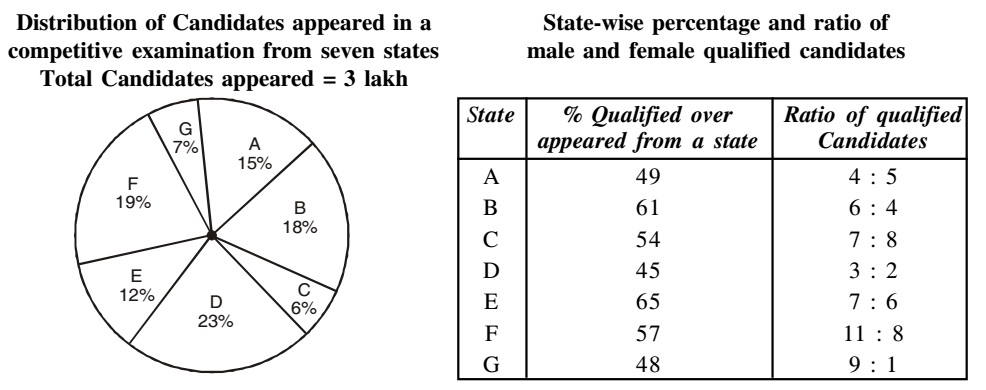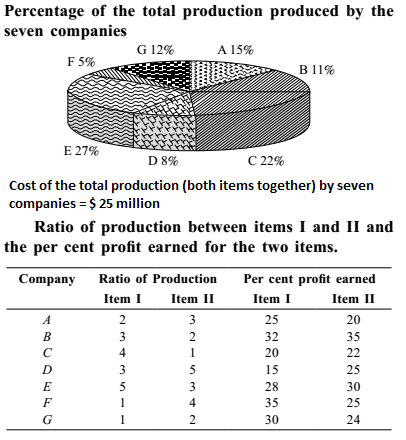## Mixed Graph

#### Data Interpretation

Direction: Study the following graph and table carefully and answer the questions given below it.1. Which of the following pair of states have equal number of qualified male candidates ?

1. Qualified Male Candidates from different states
A → 4/9 x 49/100 x 15/100 x 300000 = 9800
B → 6/10 x 61/100 x 18/100 x 300000 = 19764
C → 3/5 x 45/100 x 23/100 x 300000 = 18630
E → x 7/13 x 65/100 x 12/100 x 300000 = 12600
F → 11/19 x 57/100 x 19/100 x 300000 = 18810
G → 9/20 x 48/100 x 7/100 x 300000 = 4536
Hence, State C and G have equal number of qualified male candidates

##### Correct Option: D

Qualified Male Candidates from different states
A → 4/9 x 49/100 x 15/100 x 300000 = 9800
B → 6/10 x 61/100 x 18/100 x 300000 = 19764
C → 3/5 x 45/100 x 23/100 x 300000 = 18630
E → x 7/13 x 65/100 x 12/100 x 300000 = 12600
F → 11/19 x 57/100 x 19/100 x 300000 = 18810
G → 9/20 x 48/100 x 7/100 x 300000 = 4536
Hence, State C and G have equal number of qualified male candidates

1. What is the percentage of candidates qualified from states 'A' and 'C' together of the total candidates appeared?

1. Number of Candidates qualified from States A and C together
= 49/100 x 15/100 x 300000 + 61/100 x 18/100 x 300000
= 22050 + 32940 = 54990
Required percentage = 54990/300000 x 100 = 18.33%

##### Correct Option: B

Number of Candidates qualified from States A and C together
= 49/100 x 15/100 x 300000 + 61/100 x 18/100 x 300000
= 22050 + 32940 = 54990
Required percentage = 54990/300000 x 100 = 18.33%

Direction: Seven companies A, B, C, D, E, F and G are engaged in production of 2 items I and II. The comparative data about production of these items by the 7 companies is given in the following graph and Table. Study them carefully and answer the questions given below.1. Cost of production of item I by company F is what percent of the cost of production of item II by company D?

1.  Required % = Cost of production of item I by company F x 100 % cost of production of item II by company D

##### Correct Option: E

Cost of production of item I by company F

 = 1 x 5 x 25 = 0.25 million 5 100

Cost of production of item II by company D
 = 5 x 8 x 25 = 1.25 million 8 100

 Required % = Cost of production of item I by company F x 100 % cost of production of item II by company D

 = 0.25 x 100 = 20% 1.25

1. What is the ratio of the cost of production of item I by company A to the cost of production of item I by company D?

1. Required Ratio = cost of production of item I by company A : cost of production of item I by company D

##### Correct Option: C

Given :- Cost of production of item I by company A

 = 2 x 15 x 25 = 1.50 million 5 100

Cost of production of item I by company D
 = 3 x 8 x 25 = 0.75 million 8 100

Required Ratio = cost of production of item I by company A : cost of production of item I by company D
= 1.50 : 0.75 = 2 : 1

1. The cost of production of both items together by company E is equal to the total cost of production of both items together by which of the two companies?

1. From above given data ,
Since , The cost of production of both items together by company E is equal to the total cost of production of both items together by C and F of the two companies.
Required answer will be C and F .

##### Correct Option: D

From above given data ,
Since , The cost of production of both items together by company E is equal to the total cost of production of both items together by C and F of the two companies.
Required answer will be C and F .